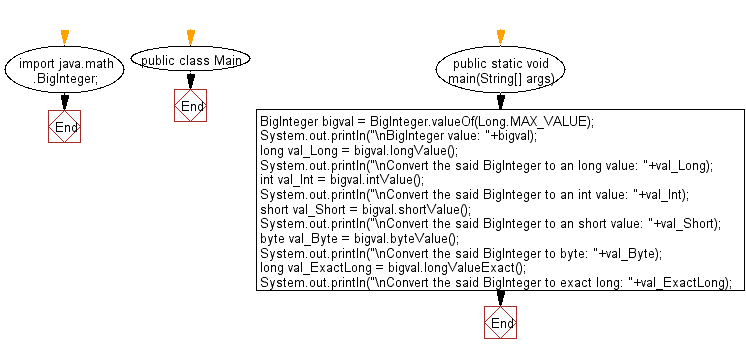﻿ Java exercises: Extract the primitive type value from a given BigInteger value - w3resource# Java Data Type Exercises: Extract the primitive type value from a given BigInteger value

## Java Data Type: Exercise-14 with Solution

Write a Java program to extract the primitive type value from a given BigInteger value.

A primitive type is predefined by the language and is named by a reserved keyword. Primitive values do not share state with other primitive values. The eight primitive data types supported by the Java programming language are byte, short, int, long, float, double, Boolean and char. BigInteger() translates the sign-magnitude representation of a BigInteger into a BigInteger. The sign is represented as an integer signum value: -1 for negative, 0 for zero, or 1 for positive. The magnitude is a byte array in big-endian byte-order: the most significant byte is in the zeroth element. A zero-length magnitude array is permissible, and will result in a BigInteger value of 0, whether signum is -1, 0 or 1.

Sample Solution:

Java Code:

``````import java.math.BigInteger;
public class Main {
public static void main(String[] args) {
BigInteger bigval = BigInteger.valueOf(Long.MAX_VALUE);
System.out.println("\nBigInteger value: "+bigval);
long val_Long = bigval.longValue();
System.out.println("\nConvert the said BigInteger to an long value: "+val_Long);
int val_Int = bigval.intValue();
System.out.println("\nConvert the said BigInteger to an int value: "+val_Int);
short val_Short = bigval.shortValue();
System.out.println("\nConvert the said BigInteger to an short value: "+val_Short);
byte val_Byte = bigval.byteValue();
System.out.println("\nConvert the said BigInteger to byte: "+val_Byte);
long val_ExactLong = bigval.longValueExact();
System.out.println("\nConvert the said BigInteger to exact long: "+val_ExactLong);
}
}
```
```

Sample Output:

```BigInteger value: 9223372036854775807

Convert the said BigInteger to an long value: 9223372036854775807

Convert the said BigInteger to an int value: -1

Convert the said BigInteger to an short value: -1

Convert the said BigInteger to byte: -1

Convert the said BigInteger to exact long: 9223372036854775807
```

Flowchart:Java Code Editor:

Improve this sample solution and post your code through Disqus

What is the difficulty level of this exercise?

﻿

## Java: Tips of the Day

Checks if a string is upper case:

```public static boolean isUpperCase(String input) {
return Objects.equals(input, input.toUpperCase());
}
```

Ref: https://bit.ly/39Hpo84